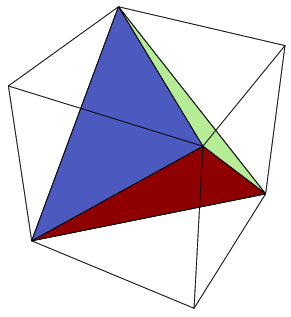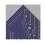# Counting triangles in squares in 7 Dimensions## In $\text{3D}$ it's possible to make a regular tetrahedron with integer coordinates that all lie on the vertices of a cube. Note that the analogous phenomena is not possible in $2$ dimensions. That is, we cannot create an equilateral triangle with co-ordinates that all lie on a square.

In another problem we showed an analogue of this occurs in infinitely many higher dimensions. Ie It is possible to make a regular n-simplex who's vertices all lie on the vertices of a regular n-hypercube. Specifically, one can show that this happens for 7-simplices and 7-hypercubes. I have 2 questions that I have tried to answer but would like to see if anyone gets the same result. SO here are the questions.

1. How many distinct 7-simplices that have vertices on a 7-hypercube all share at least one common vertex?

2. How many distinct 7-simplices in total have vertices on a common 7-hypercube?

3. What's the size of an orbit of a single 7-simplex in the set of all the 7-simplices under the group of the symmetries of the 7-hypercube?

## I have tried to answer all of these questions but if you don't want to be influenced by these (as they may be wrong) I will put these in the comments.

Details and Assumptions:

• A regular $n$-dimensional simplex in $\mathbb{Z}^n$ has $n+1$ vertices that are all an equal distance apart. (It's like an $n$-dimensional version of an equilateral triangle!)

• Here is the Wikipedia article on hypercubes. (It's like an $n$-dimensional version of a square!)Note by Roberto Nicolaides
4 years, 1 month ago

This discussion board is a place to discuss our Daily Challenges and the math and science related to those challenges. Explanations are more than just a solution — they should explain the steps and thinking strategies that you used to obtain the solution. Comments should further the discussion of math and science.

When posting on Brilliant:

• Use the emojis to react to an explanation, whether you're congratulating a job well done , or just really confused .
• Ask specific questions about the challenge or the steps in somebody's explanation. Well-posed questions can add a lot to the discussion, but posting "I don't understand!" doesn't help anyone.
• Try to contribute something new to the discussion, whether it is an extension, generalization or other idea related to the challenge.

MarkdownAppears as
*italics* or _italics_ italics
**bold** or __bold__ bold
- bulleted- list
• bulleted
• list
1. numbered2. list
1. numbered
2. list
Note: you must add a full line of space before and after lists for them to show up correctly
paragraph 1paragraph 2

paragraph 1

paragraph 2

[example link](https://brilliant.org)example link
> This is a quote
This is a quote
    # I indented these lines
# 4 spaces, and now they show
# up as a code block.

print "hello world"
# I indented these lines
# 4 spaces, and now they show
# up as a code block.

print "hello world"
MathAppears as
Remember to wrap math in $$ ... $$ or $ ... $ to ensure proper formatting.
2 \times 3 $2 \times 3$
2^{34} $2^{34}$
a_{i-1} $a_{i-1}$
\frac{2}{3} $\frac{2}{3}$
\sqrt{2} $\sqrt{2}$
\sum_{i=1}^3 $\sum_{i=1}^3$
\sin \theta $\sin \theta$
\boxed{123} $\boxed{123}$

Sort by:

POSSIBLE SPOILERS :

Answers I got for each question were 5,80,16 respectively for questions 1,2 and 3. If I have time later and anyone asks I'll write down how I got these numbers.

- 4 years, 1 month ago

For question 1, I'm getting an answer of more than 5. However, if it was assumed that all the simplexes would only intersect at 1 vertex, then the answer would be 5, which isn't true (I'm not sure if you made the same assumption as me). What I did was to consider the Hadamard Matrix of order 8. Up to equivalence, it is known that there is only 1 unique Hadamard Matrix for order 8. So to find all the 7-simplex, we only need to consider the rearrangement of the rows for the Hadamard Matrix given by Michael here, which is

${H}_{2}=\begin{pmatrix} 0 & 0 & 0 & 0 & 0 & 0 & 0 & 0 \\ 0 & 0 & 1 & 1 & 0 & 0 & 1 & 1 \\ 0 & 1 & 0 & 1 & 0 & 1 & 0 & 1 \\ 0 & 1 & 1 & 0 & 0 & 1 & 1 & 0 \\ 0 & 0 & 0 & 0 & 1 & 1 & 1 & 1 \\ 0 & 0 & 1 & 1 & 1 & 1 & 0 & 0 \\ 0 & 1 & 0 & 1 & 1 & 0 & 1 & 0 \\ 0 & 1 & 1 & 0 & 1 & 0 & 0 & 1 \end{pmatrix}$

From here, it is easy to find more than 5 solutions.

- 4 years, 1 month ago

Julian, I think this is a wonderful observation! Thanks so much :)

Update : Found my mistake, I now make it our to be 30 sharing at least one common vertex and 480 simplices in total with 16 still being in a common orbit!

- 4 years, 1 month ago

How did you get 30 though? As of right now the only method I see consists of tedious counting...

- 4 years, 1 month ago

It was a bit of a tedious counting argument using the fact that every hadamard matrix is generated by 3 linear independents Row vectors. So it came down to counting the number of ways we can create these tripples. Let me know if you want me to try write this down explicitly :) I realise I divided by an unnessary factor of 3! = 6 before. The same question seems exceptionally interesting in 11 dimensions!!

- 4 years, 1 month ago

I don't really understand. What construction did you use that requires the 3 row vectors? By the way, I tried to find more 7-simplexes with different lengths and proved that there aren't anymore apart from those described by Hadamard matrixes.

- 4 years, 1 month ago

I did not explain myself well at all, apologies. Maybe I can find some time this weekend to write down what I did rigorously and explicitly.

Nice result checking for other lengths! I expect we can prove that they must all be of this type (eg a fixed amount of 1s in each row given the dimension) by looking at two different formulas for the hypervolume of an n-simplex!

I think this is a super interesting problem to look at it in general- in particular looking at the groups acting on the set of all the simplices in the case where n=11. I suspect one can derive an interesting construction of some of the sporadic simple groups by looking at these simplices in different dimensions!

Glad that you seem to find the problem interesting too! I think I once gave a talk on this and will have the slides somewhere - let me know if you want a copy!

- 4 years, 1 month ago

H'okay, where can you send me?

- 4 years, 1 month ago

I suppose I could send you an email via my university email or send you a copy via drop box? Is there a discrete way to send info such as emails via Brilliant - something like private messaging? I don't know if there is a way to do this all within Brilliant.

I will also aim to start writing a complete account of what I did, dotting the is and crossing the ts this weekend ( I think it'll be good to clarify some thoughts in my head also!)

- 4 years, 1 month ago

In the case where n=11, there are also no 11-simplices that can't be expressed with Hadamard. As of now, I have yet to find a method to prove for all $n=4k-1$. My bounding method only works for $n<12$. I'll work on it during the weekend as that's when my exams end.

- 4 years, 1 month ago

Interesting, I'd love to see this bounding method. I've dropped you an email so feel free to delete this comment if/when you receive it :)

- 4 years, 1 month ago